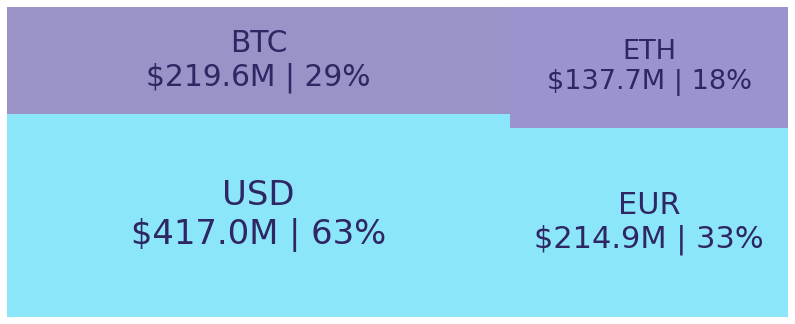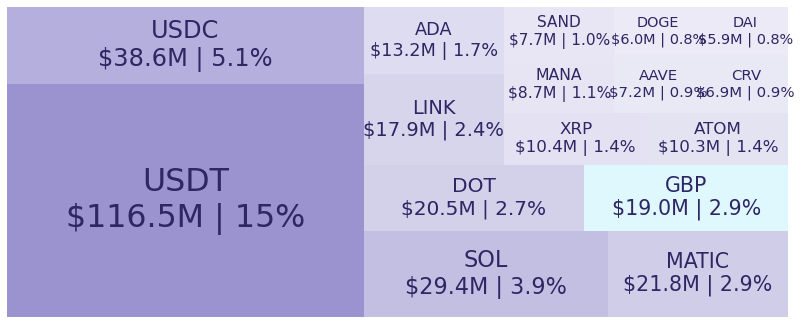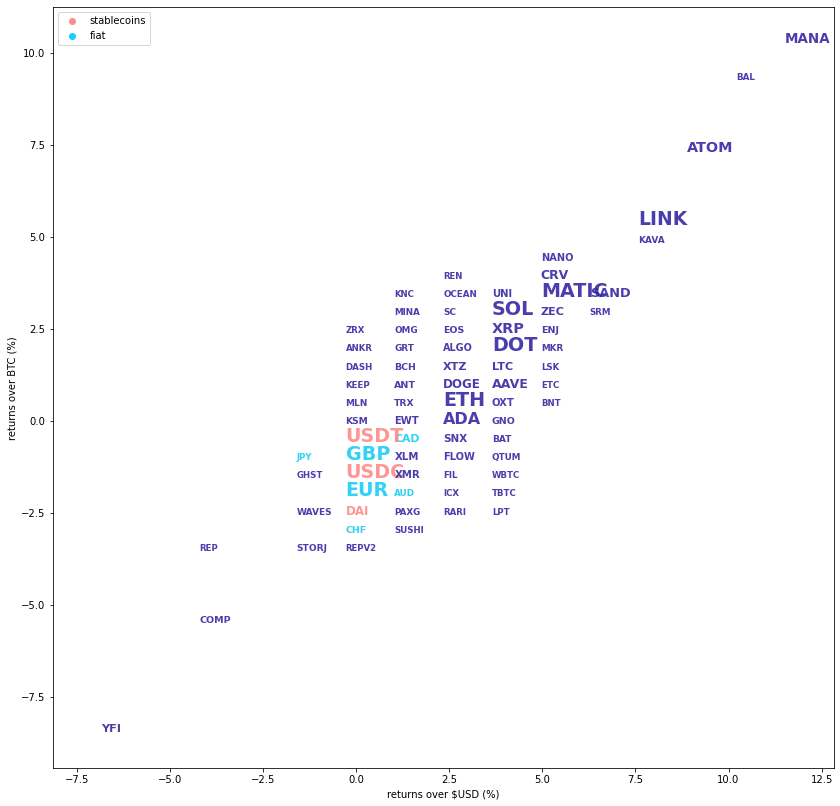# Kraken Daily Market Report for December 18 2021

## Overview

• Total spot trading volume at $707.4 million, the 30 day average is$1.5 billion.
• Total futures notional at $249.3 million. • The top traded coins were the same as yesterday: Bitcoin (+1.5%), Ethereum (2.2%), Tether (0%), USDC (0%), and Solana (+4.0%). • Strong returns from Mana (+12%), Balancer (+10%), and Cosmos (+9.3%). December 18, 2021$707.4M traded across all markets today
Crypto, EUR, USD, JPY, CAD, GBP, CHF, AUD
BTC
$46864. ↑1.5%$219.6M
ETH
$3961.3 ↑2.2%$137.7M
USDT
$1.0004 ↑0.01%$116.5M
USDC
$1.0001 ↑0.0%$38.6M
SOL
$182.62 ↑4.0%$29.4M
MATIC
$2.2339 ↑5.0%$21.8M
DOT
$25.515 ↑3.5%$20.5M
$19.539 ↑7.0%$17.9M
$1.2426 ↑2.0%$13.2M
XRP
$0.8267 ↑3.5%$10.4M
ATOM
$23.165 ↑9.3%$10.3M
MANA
$3.4368 ↑12%$8.71M
SAND
$5.1689 ↑5.9%$7.7M
AAVE
$193.2 ↑3.2%$7.18M
CRV
$4.5169 ↑5.3%$6.91M
DOGE
$0.1723 ↑2.0%$5.99M
DAI
$1.0000 ↑0.01%$5.87M
LUNA
$74.62 ↑14%$5.68M
YFI
$31113. ↓7.0%$5.01M
XTZ
$4.2463 ↑2.5%$4.75M
LTC
$148.88 ↑3.4%$4.74M
ZEC
$157.80 ↑5.1%$4.64M
SNX
$5.185 ↑1.8%$3.22M
XLM
$0.2560 ↑0.6%$2.97M
XMR
$181.62 ↓0.07%$2.88M
UNI
$15.585 ↑3.8%$2.72M
OXT
$0.3624 ↑2.4%$2.58M
EWT
$10.77 ↑0.9%$2.52M
FLOW
$8.493 ↑2.2%$2.38M
NANO
$3.3751 ↑5.5%$2.29M
ALGO
$1.3791 ↑2.3%$1.93M
COMP
$204.72 ↓4.2%$1.8M
ENJ
$2.408 ↑4.6%$1.79M
ANT
$5.8416 ↑2.4%$1.73M
EOS
$3.2462 ↑2.9%$1.59M
GNO
$446.82 ↑2.5%$1.59M
BCH
$436.44 ↑2.8%$1.36M
LRC
$2.0696 ↑1.4%$1.17M
TRX
$0.0804 ↑1.2%$1.14M
KSM
$280.28 ↑1.1%$1.14M
SHIB
$3.146e ↑0.9%$1.08M
STORJ
$1.7836 ↓1.5%$967K
BAT
$1.1772 ↑2.4%$946K
WAVES
$17.310 ↓0.8%$924K
MLN
$85.363 ↑0.3%$791K
KAVA
$3.782 ↑7.1%$784K
SC
$0.0154 ↑2.5%$749K
FIL
$36.339 ↑1.9%$556K
GRT
$0.6053 ↑2.8%$554K
OMG
$6.0519 ↑1.9%$523K
QTUM
$9.2972 ↑2.6%$509K
MKR
$2479.3 ↑3.9%$475K
$15.437 ↓1.2%$467K
BAL
$18.09 ↑10%$465K
MINA
$3.23 ↑2.2%$461K
CTSI
$0.6614 ↑2.3%$432K
DYDX
$8.1468 ↑1.4%$406K
MOVR
$191.53 ↑1.8%$404K
PAXG
$1804.0 ↑0.0%$373K
PERP
$9.396 ↑1.9%$370K
AXS
$96.5 ↑2.2%$361K
OCEAN
$0.79 ↑2.3%$345K
KILT
$4.12 ↓3.7%$340K
KEEP
$0.6027 ↑1.5%$330K
SUSHI
$5.6 ↑1.1%$312K
DASH
$129.71 ↑1.1%$281K
SRM
$3.485 ↑5.5%$264K
ICX
$1.2238 ↑1.9%$251K
REP
$17.38 ↓3.8%$243K
ETC
$35.114 ↑2.3%$234K
INJ
$8.137 ↑1.5%$231K
LSK
$2.4045 ↑5.2%$230K
CQT
$0.583 ↑4.9%$201K
RARI
$13.7 ↑1.3%$192K
MIR
$2.557 ↑3.2%$190K
ANKR
$0.1024 ↑1.3%$165K
1INCH
$2.465 ↑0.8%$150K
PHA
$0.361 ↑3.2%$132K
GHST
$2.3872 ↓0.4%$132K
CHZ
$0.2726 ↑2.6%$128K
REN
$0.515 ↑2.3%$124K
SDN
$1.5775 ↑2.6%$85.0K
KNC
$1.3284 ↑2.1%$79.2K
LPT
$36.41 ↑2.1%$64.8K
RAY
$7.371 ↑2.8%$55.3K
WBTC
$46822. ↑1.7%$52.5K
REPV2
$16.866 ↑0.09%$35.1K
ZRX
$0.7859 ↑1.9%$34.1K
KAR
$3.681 ↑0.7%$29.2K
BNC
$1.72 ↓1.7%$16.1K
OXY
$1.197 ↑3.0%$12.0K
OGN
$0.6038 ↑1.8%$9.43K
BAND
$5.199 ↑4.0%$7.48K
TBTC
$47052. ↑2.0%$6.64K
BNT
$3.285 ↑3.6%$5.91K

#####################. Trading Volume by Asset. ##########################################

The figures below break down the trading volume of the largest, mid-size, and smallest assets. Cryptos are in purple, fiats are in blue. For each asset, the chart contains the daily trading volume in USD, and the percentage of the total trading volume. The percentages for fiats and cryptos are treated separately, so that they both add up to 100%.

Figure 1: Largest trading assets: trading volume (measured in USD) and its percentage of the total trading volume (December 18 2021)Figure 2: Mid-size trading assets: (measured in USD) (December 18 2021)###########. Daily Returns. #################################################

## Daily Returns %

Figure 3: Returns over USD and XBT. Relative volume and return size is indicated by the size of the font. (December 18 2021)###########. Disclaimer #################################################

The values generated in this report are from public market data distributed from Kraken WebSockets api. The total volumes and returns are calculated over the reporting day using UTC time.